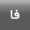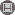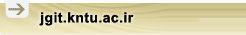[Home ] [Archive]   [ فارسی ]Volume 7, Issue 3 (12-2019)jgit 2019, 7(3): 213-230 Back to browse issues page
Estimation of Tree Biomass at Individual tree, Sample plot and Hybrid Level using Drone Images
Tarbiat Modares University
Abstract:   (261 Views)
Two-dimensional image conversion algorithms to 3D data create the hope that the structural properties of trees can be extracted through these images. In this study, the accuracy of biomass estimation in tree, plot, and hybrid levels using UAVs images was investigated. In 34.8 ha of Sisangan Forest Park, using a quadcopter, 854 images from an altitude of 100 meters above ground were acquired. SFM algorithm was applied to produce 3D data and the height of the trees was extracted. 28 samples with 30×30 m dimension were collected and the height and the diameter at the breast height were measured and the biomass was calculated based on a general allometric equation. In order to estimate the biomass at plot-level, the height metrics were extracted from the point cloud. For tree-level, the biomass of each tree was modeled based on the height value derived from CHM for each tree. In hybrid-level, sum of estimated biomass of trees in tree-level was used as the predictor in modeling. The accuracy and precision of the estimates were evaluated using relative bias (rBias), relative root mean square error (rRMSE), and adjusted r square (r2). rRMSE for biomass estimation in Buxus hyrcana, Carpinus betulus, Parottia persica, and other species were 17.56, 7.11, 14.67 and 22.73 percent, respectively. For plot level and hybrid level, rRMSE were 58 and 47 percent, respectively. Based on the result, the most precise approach for biomass estimation is hybrid level and the precision of the estimate is appropriate for overall assessment of forest stands, not for management planning.
Keywords: UAV, Sisangan, Crown Height Model, Above Ground Biomass, Digital Terrain Model.
Type of Study: Research | Subject: RS
Received: 2018/07/30 | Accepted: 2019/05/25 | Published: 2019/12/21
Send email to the article author